# 1 Introduction

Data visualisation is a powerful tool used for data analysis and exploration in many fields. Genomics data analysis is one of these fields where good visualisation tools can be of great help. The aim of karyoploteR is to offer the user an easy way to plot data along the genome to get broad wide view where it is possible to identify genome wide relations and distributions.

karyoploteR is based on base R graphics and mimicks its interface. You first create a plot with plotKaryotype and then sequentially call a number of functions (kpLines, kpPoints, kpBars…) to add data to the plot.

karyoploteR is a plotting tool and only a plotting tool. That means that it is not able to download or retrieve any data. The downside of this is that the user is responsible of getting the data into R. The upside is that it is not tied to any data provider and thus can be used to plot genomic data coming from anywhere. The only exception to this are the ideograms cytobands, that by default are plotted using predownloaded data from UCSC.

# 2 Quick Start

The basic idea behind karyoploteR has been to create a plotting system inspired by the R base graphics. Therefore, the basic workflow to create a karyoplot is to start with an empty plot with no data apart from the ideograms themselves using plotKaryotype and then add the data plots as required. To add the data there are functions based on the R base graphics low-level primitives -e.g kpPoints, kpLines, kpSegments, kpRects… - that can be used to plot virtually anything along the genome and other functions at a higher level useful to plot more specific genomic data types -e.g. kpPlotRegions, kpPlotCoverage…-.

This is a first simple example plotting a set of regions representing copy-number gains and losses using the kpPlotRegions function:

  gains <- makeGRangesFromDataFrame(data.frame(chr=c("chr1", "chr5", "chr17", "chr22"), start=c(1, 1000000, 4000000, 1),
end=c(5000000, 3200000, 80000000, 1200000)))
losses <- makeGRangesFromDataFrame(data.frame(chr=c("chr3", "chr9", "chr17"), start=c(80000000, 20000000, 1),
end=c(170000000, 30000000, 25000000)))
kp <- plotKaryotype(genome="hg19")
kpPlotRegions(kp, gains, col="#FFAACC")
kpPlotRegions(kp, losses, col="#CCFFAA")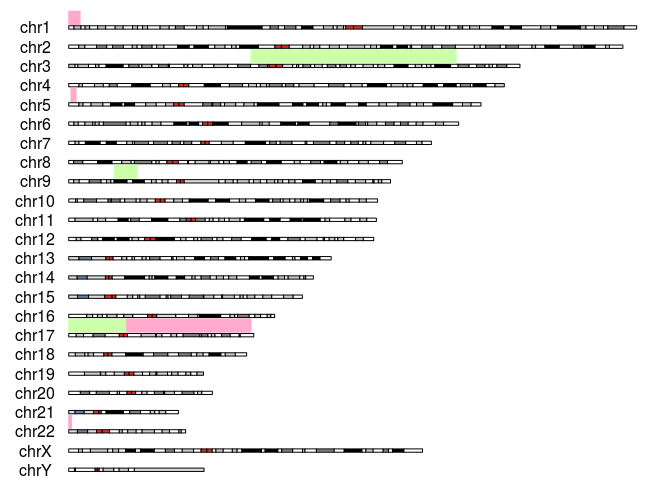As you can see, the plotKaryotype returns a KaryoPlot object that has to be passed to any subsequent plot call.

plotKaryotype accepts a number of parameters but the most commonly used are genome, chromosomes and plot.type. The genome and chromosomes are used to specify the genome to be plotted (defaults to hg19 and which chromosomes to plot (defaults to canonical). The plot.type parameter is used to select between different modes of adding data to the genome (above, below or on the ideograms).

For example, to create a plot of the mouse genome with data above the ideograms we would use this:

  kp <- plotKaryotype(genome="mm10", plot.type=1, main="The mm10 genome")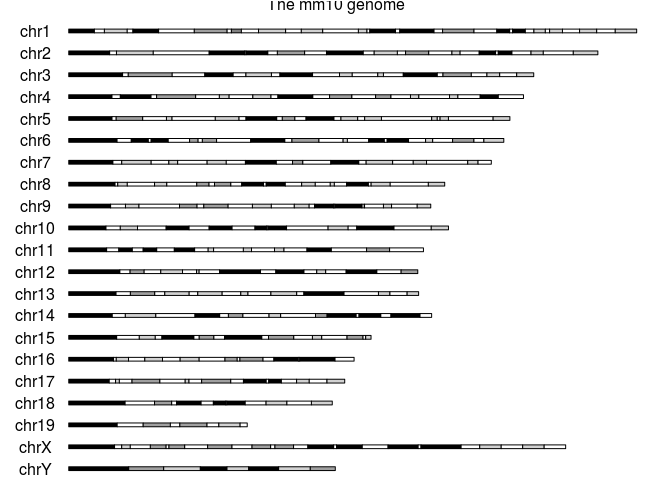And to plot the first thee chromosomes of the hg19 human genome assembly with data above and below them:

  kp <- plotKaryotype(genome="hg19", plot.type=2, chromosomes=c("chr1", "chr2", "chr3"))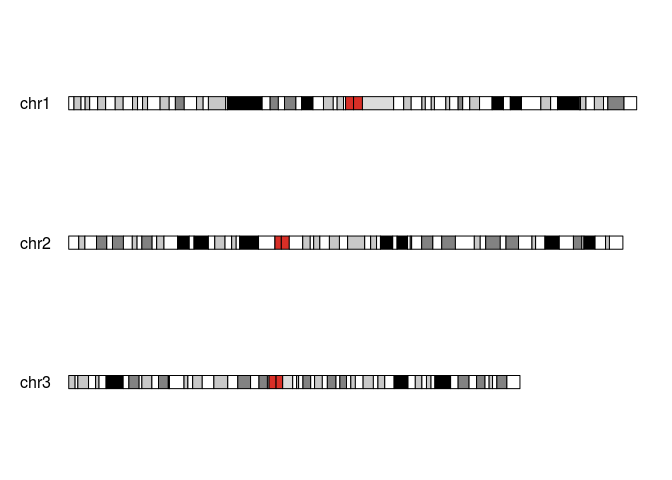All low-level plotting functions share a similar interface, and in general, they accept the standard R plotting parameters (lwd, cex, pch, etc…). The simplest way (althought not always the most convenient) is to treat them as the equivalent R base plotting functions with an additional chr parameter. As an example, we can create a set of random 1 base regions (using regioneR createRandomRegions) and add a random y value to them:

  rand.data <- createRandomRegions(genome="hg19", nregions=1000, length.mean=1, length.sd=0,
rand.data <- toDataframe(sort(rand.data))
rand.data <- cbind(rand.data, y=runif(n=1000, min=-1, max=1))

#Select some data points as "special ones"
sel.data <- rand.data[c(7, 30, 38, 52),]
head(rand.data)
##    chr    start      end           y
## 1 chr1  3534489  3534489  0.37182379
## 2 chr1  9765550  9765550 -0.75039143
## 3 chr1 10660469 10660469 -0.62290400
## 4 chr1 10918836 10918836  0.50535078
## 5 chr1 13722678 13722678 -0.05097132
## 6 chr1 17247042 17247042  0.84357538

And then plot them in different ways.

  kp <- plotKaryotype(genome="hg19", plot.type=2, chromosomes=c("chr1", "chr2", "chr3"))

kpDataBackground(kp, data.panel = 1, r0=0, r1=0.45)
kpAxis(kp, ymin=-1, ymax=1, r0=0.05, r1=0.4, col="gray50", cex=0.5)
kpPoints(kp, chr=rand.data$chr, x=rand.data$start, y=rand.data$y, ymin=-1, ymax=1, r0=0.05, r1=0.4, col="black", pch=".", cex=2) kpPoints(kp, chr=sel.data$chr, x=sel.data$start, y=sel.data$y,
ymin=-1, ymax=1, r0=0.05, r1=0.4, col="red")
kpText(kp, chr=sel.data$chr, x=sel.data$start, y=sel.data$y, ymin=-1, ymax=1, r0=0.05, r1=0.4, labels=c("A", "B", "C", "D"), col="red", pos=4, cex=0.8) #Upper part: data.panel=1 kpDataBackground(kp, data.panel = 1, r0=0.5, r1=1) kpAxis(kp, ymin=-1, ymax=1, r0=0.5, r1=1, col="gray50", cex=0.5, numticks = 5) kpAbline(kp, h=c(-0.5, 0, 0.5), col="gray50", ymin=-1, ymax=1, r0=0.5, r1=1) kpLines(kp, chr=rand.data$chr, x=rand.data$start, y=rand.data$y,
col="#AA88FF", ymin=-1, ymax=1, r0=0.5, r1=1)
#Use kpSegments to add small tic to the line
kpSegments(kp, chr=rand.data$chr, x0=rand.data$start, x1=rand.data$start, y0=rand.data$y-0.1, y1=rand.data$y+0.1, col="#8866DD", ymin=-1, ymax=1, r0=0.5, r1=1) #Plot the same line but inverting the data by pssing a r0>r1 kpLines(kp, chr=rand.data$chr, x=rand.data$start, y=rand.data$y,
col="#FF88AA", ymin=-1, ymax=1, r0=1, r1=0.5)

#Lower part: data.panel=2
kpDataBackground(kp, r0=0, r1=0.29, color = "#EEFFEE", data.panel = 2)
kpAxis(kp, col="#AADDAA", ymin=-1, ymax=1, r0=0, r1=0.29, data.panel = 2,
numticks = 2, cex=0.5, tick.len = 0)
kpAbline(kp, h=0, col="#AADDAA", ymin=-1, ymax=1, r0=0, r1=0.29, data.panel = 2)
kpBars(kp, chr=rand.data$chr, x0=rand.data$start, x1=rand.data$end, y1 = rand.data$y,

kpDataBackground(kp, r0=0.34, r1=0.63, color = "#EEEEFF", data.panel = 2)
kpAxis(kp, col="#AAAADD", ymin=-1, ymax=1, r0=0.34, r1=0.63, data.panel = 2,
numticks = 2, cex=0.5, tick.len = 0)
kpAbline(kp, h=0, col="#AAAADD", ymin=-1, ymax=1, r0=0.34, r1=0.63, data.panel = 2)
kpSegments(kp, chr=rand.data$chr, x0=rand.data$start, x1=rand.data$end, y0=rand.data$y-0.2, y1=rand.data$y, col="#AAAADD", ymin=-1, ymax=1, r0=0.34, r1=0.63, data.panel = 2, lwd=2) kpDataBackground(kp, r0=0.68, r1=0.97, color = "#FFEEEE", data.panel = 2) kpAxis(kp, col="#DDAAAA", ymin=-1, ymax=1, r0=0.68, r1=0.97, data.panel = 2, numticks = 2, cex=0.5, tick.len = 0) kpPoints(kp, chr=rand.data$chr, x=rand.data$start, y=rand.data$y,
col="#DDAAAA", ymin=-1, ymax=1, r0=0.68, r1=0.97, data.panel = 2, pch=".", cex=3)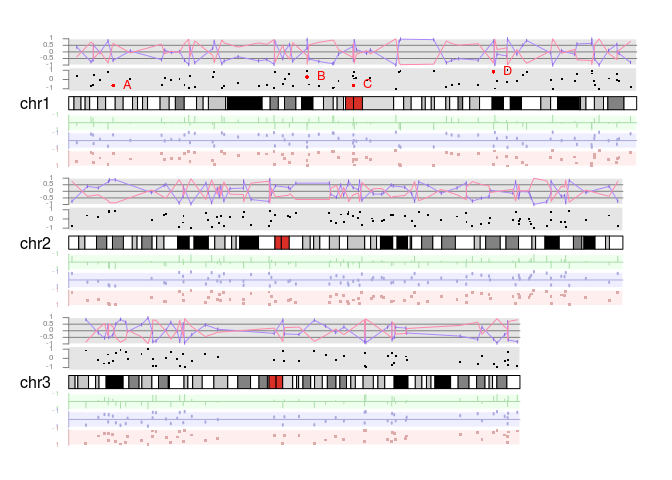The interface for the higher level plotting functions is a little different and they usually take Bioconductor objects (GRanges, etc…). As an example of these plotting functions we can create and plot 10 sets of 2000 random regions and see their coverage on the genome. In addition, we will plot the masked regions of the genomes where no random region should be.

  n <- 10
kp <- plotKaryotype(plot.type=2)
kpPlotRegions(kp, data=getGenomeAndMask("hg19")$mask, r0=0, r1=1, col="lightgray", border="lightgray") all.regions <- lapply(1:n, function(i) { filterChromosomes(createRandomRegions(nregions=2000, length.mean=100000)) }) #using invisible to ignore the return of kpPlotRegions invisible(lapply(1:n, function(i) { kpPlotRegions(kp, all.regions[[i]], r0=(1/n)*(i-1), r1=(1/n)*i) })) kpPlotCoverage(kp, data=do.call(c, all.regions), data.panel=2, col="#AAAAFF")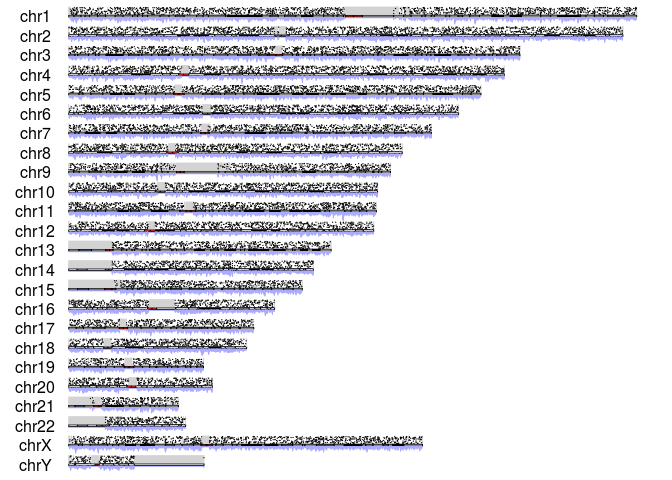# 3 Creating a karyotype plot All plots in karyoploteR start with a call to plotKaryotype. This function is used to define the desired type of plot and the basic plotting parameters, creates an empty plot with the ideograms representing the chromosomes and finally returns a KaryoPlot object that will be needed by all functions adding data into the plot. There are two main parts in the karyoplots: the ideograms and the data panels. The ideograms represent the chromosomes and are usually represented by the chromosomic cytobands. The data panels are the parts of the plot where data will be plotted. They are not marked by default (no border or background) and depending on the plot type there may be more than one per chromosome. plotKaryotype accepts a number of parameters to modify its behaviour, but by default it will create an empty karyoplot of the human genome version hg19 with a single data panel above the ideograms:  kp <- plotKaryotype()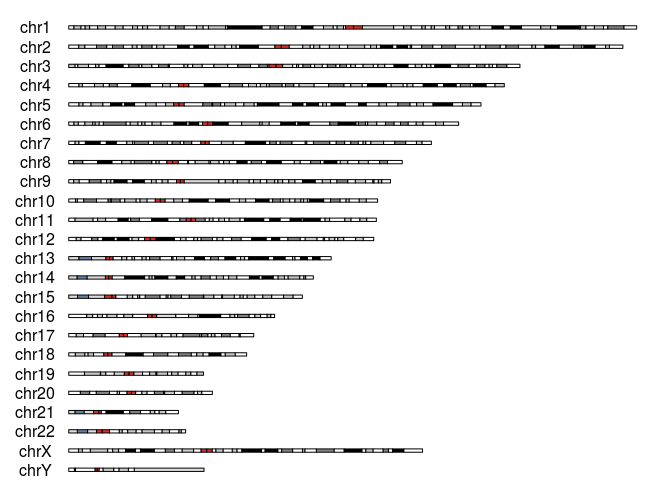In this section we will see how the different parameters of plotKarytype can be used to modify this basic plot and create plots with more data panels, for other organisms, with a different style or showing just some of the chromosomes. ## 3.1 Genomes and Chromosomes A karyoplot is a representation of a genome, and thus, one of the main parameters of plotKaryotype is genome. With it we can specify what genome we want to plot. The package includes a small cache with information on some genomes and to use it we have to specify the genome using the standard UCSC genome name (hg19, hg38, mm10, dm6, …). However, internally it can use regioneR’s getGenomeAndMask function and so we can specify the genome in any fornmat accepted by this function, including the name of any BSGenome object available in the system. If we want plotKaryotype to ignore the included cache and try to automatically get the genome information from other sources we can set use.cache to FALSE. In addition, with the chromosomes parameter, it is possible to plot only a subset of the chromosomes of the genome. The chromosomes can be specified either as a list of chromosome names or as one of the predefined sets for the available organisms: autosomal, canonical and all. By default, only canonical chromosomes are drawn. The chromosomes parameter can also be used to change the order of the chromosomes.  kp <- plotKaryotype(chromosomes=c("autosomal"))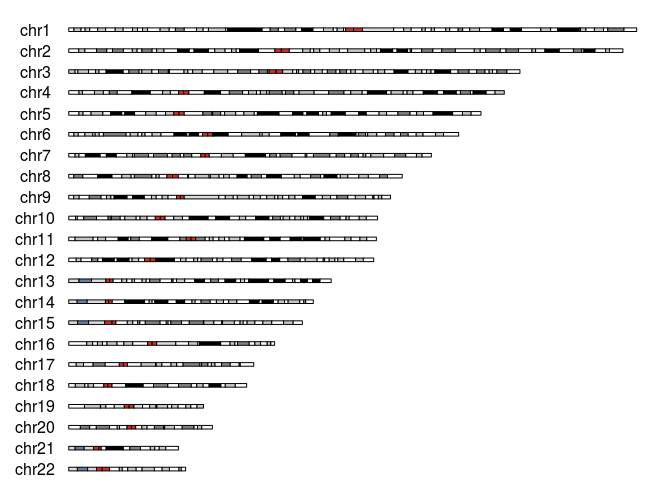kp <- plotKaryotype(genome="mm10", chromosomes=c("chr10", "chr13", "chr2"))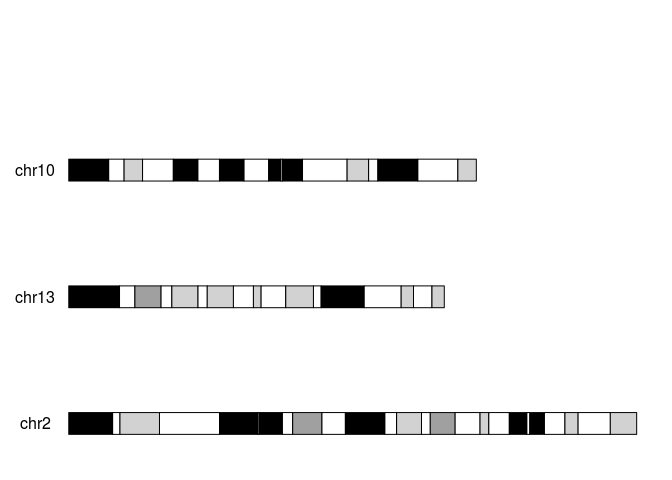## 3.2 Types of Plots There are currently 5 plot types available: • plot.type=1 A plot with stacked horizontal ideograms and a single data panel on top of them • plot.type=2 A plot with stacked horizontal ideograms and two data panels, one above and one below them • plot.type=3 A plot with horizontal ideograms all in one level, and two data panels, one above and one below them • plot.type=4 A plot with horizontal ideograms all in one level, and one data panel above them • plot.type=5 A plot with horizontal ideograms all in one level, and one data panel below them To clearly see where the data panels are and in some cases, to help the readers interpret the data it is possible to add a background to the data panels with kpDataBackground. Please take into account that the function draws a solid rectangle and so if any data was already plotted it would be no longer visible. This is plot type 1:  kp <- plotKaryotype(chromosomes = c("chr1", "chr2"), plot.type = 1) kpDataBackground(kp, data.panel = 1)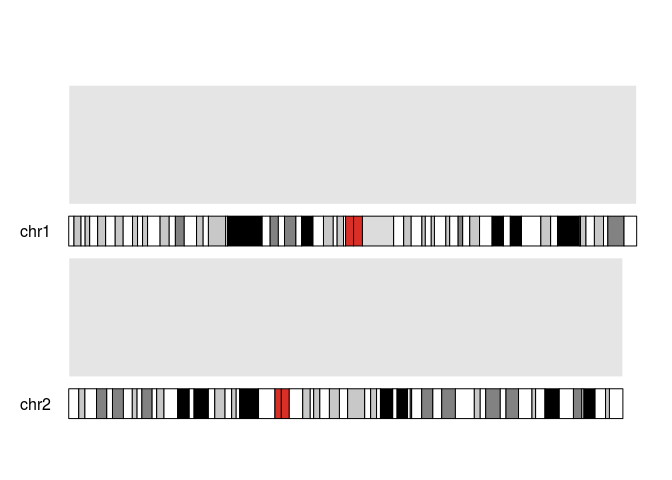This is plot type 2:  kp <- plotKaryotype(chromosomes = c("chr1", "chr2"), plot.type = 2) kpDataBackground(kp, data.panel = 1) kpDataBackground(kp, data.panel = 2)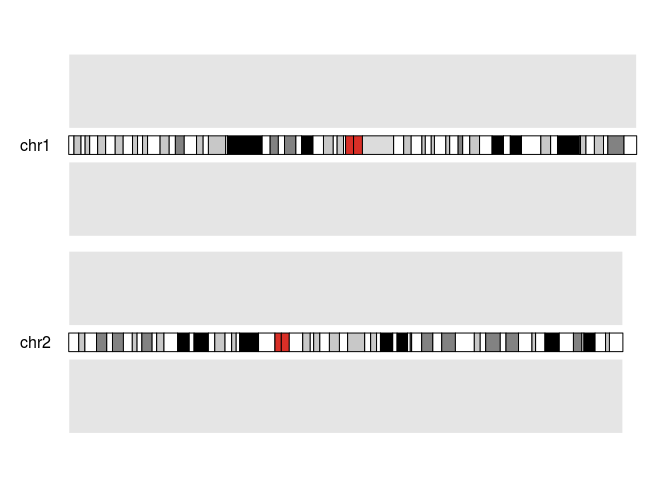This is plot type 3:  kp <- plotKaryotype(chromosomes = c("chr1", "chr2"), plot.type = 3) kpDataBackground(kp, data.panel = 1) kpDataBackground(kp, data.panel = 2)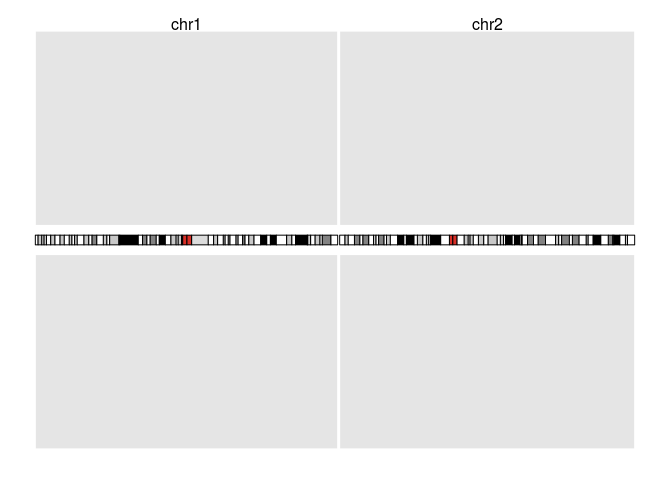This is plot type 4:  kp <- plotKaryotype(chromosomes = c("chr1", "chr2"), plot.type = 4) kpDataBackground(kp, data.panel = 1)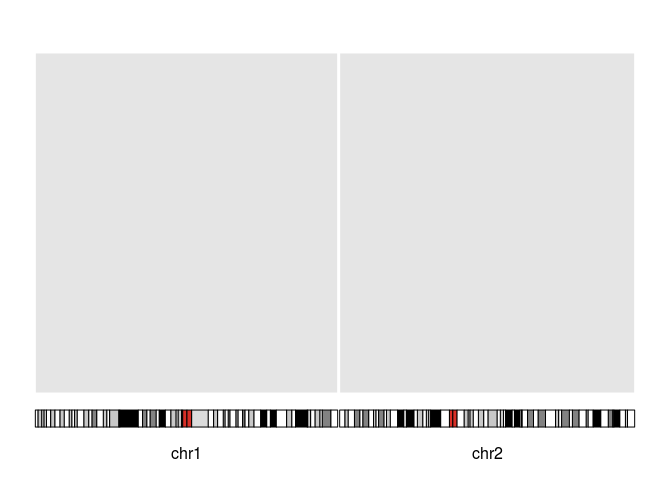And this is plot type 5:  kp <- plotKaryotype(chromosomes = c("chr1", "chr2"), plot.type = 5) kpDataBackground(kp, data.panel = 1)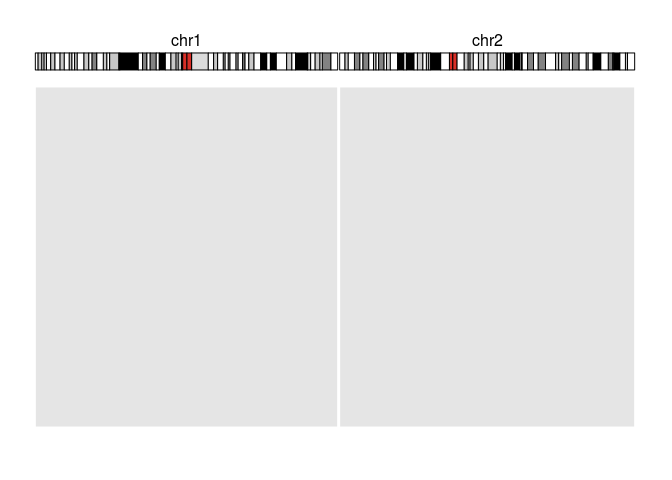## 3.3 Adding Axis In some cases, adding axis to the plots can help stablishing a context for the data. kpAxis draws an axis on the letf or right side of the data panel and it’s possible to change it’s appearance, position, labels and ticks. If combined with a kpDataBackground, its important to draw the axis after the background, so it’s not overdrawn by it.  kp <- plotKaryotype(chromosomes=c("chr1", "chr2"), plot.type=2) #data.panel=1 kpDataBackground(kp) #Default axis kpAxis(kp) #Axis on the right side of the data.panel kpAxis(kp, side = 2) #data.panel=2 kpDataBackground(kp, r1=0.47, data.panel=2) #Changing the limits and having more ticks, with a smaller font size kpAxis(kp, r1=0.47, ymin=-5000, ymax = 5000, numticks = 5, cex=0.5, data.panel=2) #and a different scale on the right kpAxis(kp, r1=0.47, ymin=-2, ymax = 2, numticks = 3, cex=0.5, data.panel=2, side=2) kpDataBackground(kp, r0=0.53, data.panel=2) #Changing the colors and labels and tick positions kpAxis(kp, r0=0.53, tick.pos = c(0.3, 0.6, 1), labels = c("A", "B", "C"), col="#66AADD", cex=0.5, data.panel=2)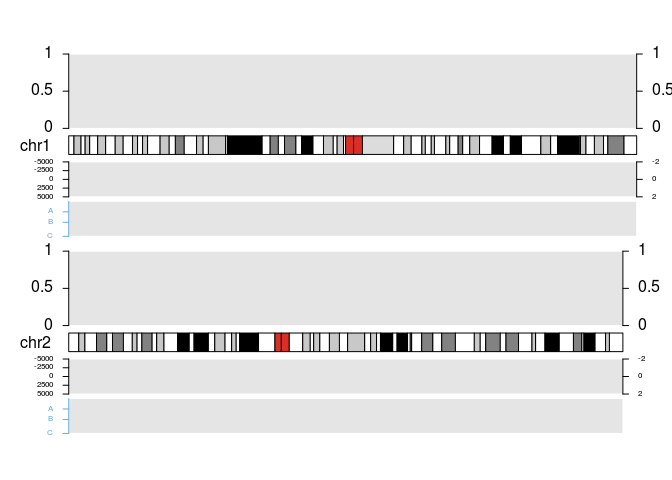## 3.4 Changing the plotting parameters In addition to changing the genomes, chromosomes, and plot types, it’s also possible to change and customize the different sizes and margins defining the look of the karyoplot. To do this we need to to provide an object with all the plotting parameters as the plot.params parameter of plotKaryotype. The easiest way to get a valid plot.params object is calling getDefaultPlotParams and modifying the response. To see the available plot params and their default values we can call plotDefaultPlotParams, which will create a plot with the representation of the plot parameters. Note that the plotting parameters plot are only implemented for plot types 1, 2 and 3. This is for plot.type=2 plotDefaultPlotParams(plot.type=2, cex=0.6)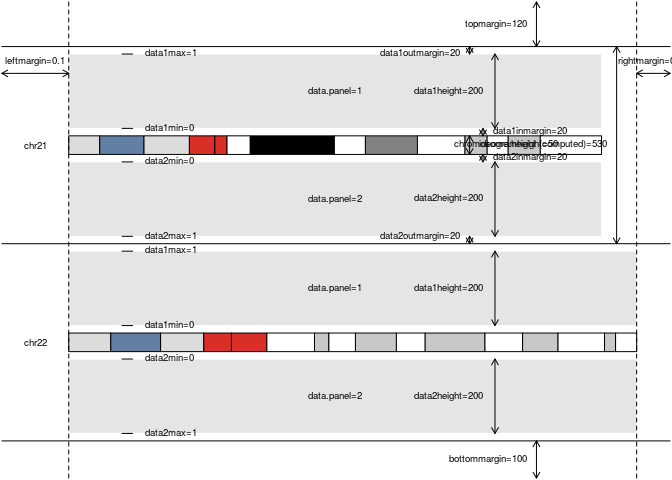And this is for plot.type=3 plotDefaultPlotParams(plot.type=3, cex=0.6)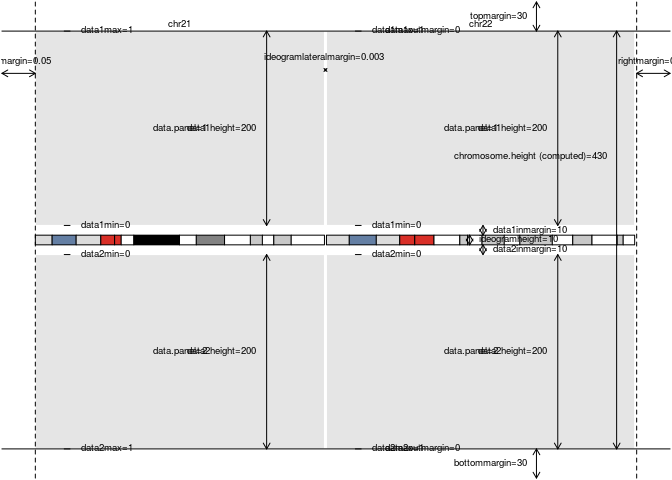Or we can change the plot parameters to create different configurations. For example, we can create a plot with a data panel larger than the other and smaller margins: plot.params <- getDefaultPlotParams(plot.type=2) plot.params$ideogramheight <- 5
plot.params$data2height <- 50 plot.params$leftmargin <- 0.05
plot.params$bottommargin <- 20 plot.params$topmargin <- 20

plotDefaultPlotParams(plot.type=2, plot.params=plot.params, cex=0.6)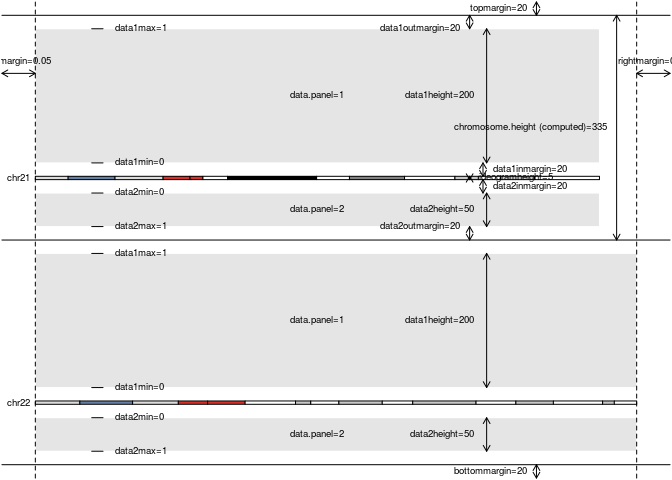Or a plot with a very small data panel above the ideogram almost touching it (for example, to mark the regions of interest) and a bigger data panel below it (for example, to plot the detailed data):

plot.params <- getDefaultPlotParams(plot.type=3)
plot.params$ideogramheight <- 50 plot.params$data1height <- 50
plot.params$data1inmargin <- 1 plot.params$data2height <- 400

plotDefaultPlotParams(plot.type = 3, plot.params=plot.params, cex=0.6)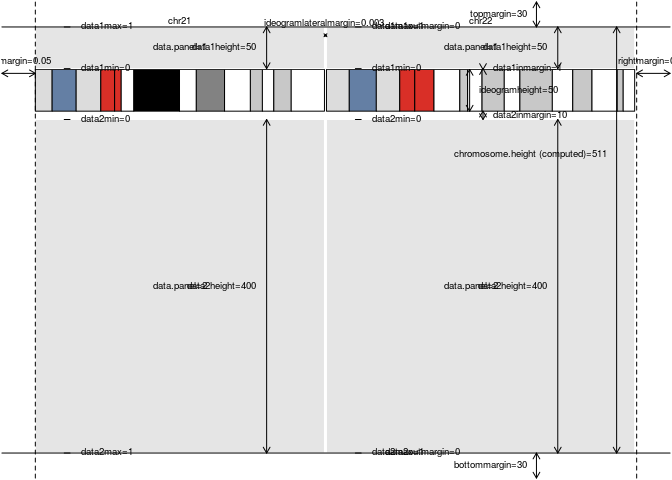After creating a plot we can add data to it using successive calls to the various data plotting functions. There are two types of data plotting functions: the low levels ones, based on the basic plotting functions from base graphics (kpPoints, kpLines, etc…) and the higher level ones, that are functions specialized in drawing certain object types such as GRanges (kpPlotRegions, kpPlotCoverage). It is perfectly possible to draw new data on top of the already plotted one in order to create combined plots.

In addition, it is be possible to create custom plotting functions based on the coordinates change function included in the karyoplot object.

## 4.1 Common Parameters

All plotting functions share a set of common parameters that define where and how data will be plotted.

r0 and r1 define the region of the data panel where the data will be plotted. They are inspired by the r0 and r1 parameters in Circos, where they define the min and max radius where the data will be confined. A value of 0 is the most proximal to the ideogram and 1 is the farthest one. In addition, if r0>r1, the data will be “flipped”, drawing larger values of y closer to the ideogram.

ymin and ymax define the minimum and maximum expected y value to be plotted. By default they are 0 and 1 but any finite number is possible. They work together with r0 and r1 to define where a data point will be drawn. In general, a data point with y=ymin will be plotted at r0 and a data point with y=ymax will be plotted at r1.

data.panel states in which data panel (if there’s more than one available) the data will be plotted. The data panel identifier depends on the plot.type.

In addition, all plotting functions accept the applicable standard R graphic parameters (lwd, col, cex, …)

## 4.2 Basic Plotting Functions

The basic or low-level plotting functions mimmick the base R plotting functions and are use to draw basic types: points, lines, segments, arrows, etc…

All basic plotting functions share a number of parameters to specify the data points. chr accepts a vector of chromosome names stating the chromosome to which data point belongs. x (or x0 and x1) are used to specify the horizontal position of the data points (or its start and end) and the units are base pairs. y (or y0 and y1) specify the value (or start and end value) of the data point and should be in the range [ymin-ymax]. All parameters are recycled if needed using the standard vector recycling rules, but in some situations this might result in strange results.

For example, to plot a point with value 0.2 in position 30Mb of chromosome 1 and a rectangle with values 0.2 to 0.4 in positions from 100Mb to 120Mb we would use:

  kp <- plotKaryotype(chromosomes=c("chr1"))
kpDataBackground(kp)
kpAxis(kp)
kpPoints(kp, chr="chr1", x=30000000, y=0.2)
kpRect(kp, chr="chr1", x0=100000000, x1=120000000, y0=0.2, y1=0.4)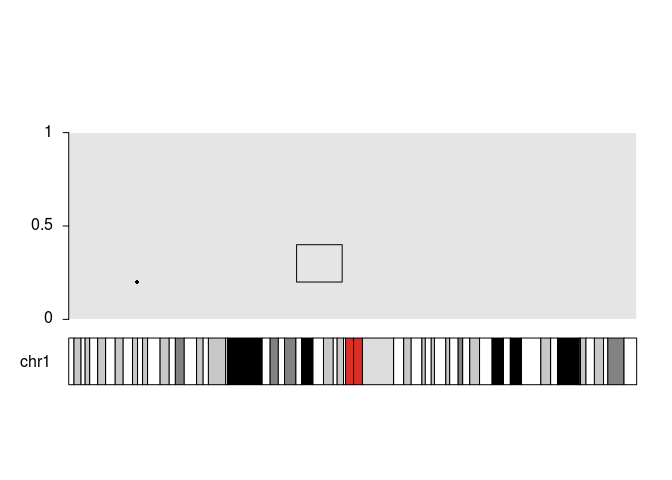Another possibility is to create a GRanges object with the data, with chr, x0 and x1 as the sequence, start and end, and one (y) or two (y0 and y1) metadata columns with the values. This GRanges object can then be passed in in the data parameter. In addition, it is possible to overrule the content of data if any of the explicit parameters is used.

  dd <- toGRanges(data.frame(chr="chr1", start=30000000, end=30000000, y=0.2))
dd2 <- toGRanges(data.frame(chr="chr1", start=100000000, end=120000000, y0=0.2, y1=0.4))

kp <- plotKaryotype(chromosomes=c("chr1"))
kpDataBackground(kp)
kpAxis(kp)
kpPoints(kp, data=dd)
kpPoints(kp, data=dd, y=0.8)
kpRect(kp, data=dd2)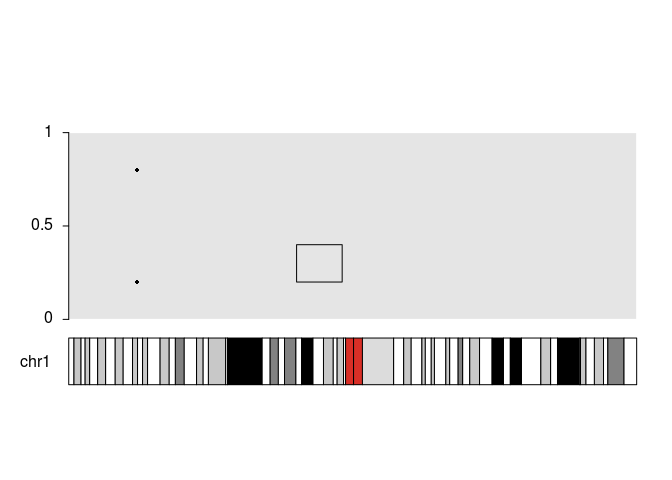These are the available basic plotting functions:

• kpAbline Draws horizontal and vertical lines spanning the whole avaliable space
• kpArrows Draws arrows. Using the code parameter, it’s possible to specify where the arrowhead should be placed.
• kpBars Draws vertical bars. If y0 is present, bars span from y0 to y1, if it’s ommited, bars span from 0 to y1
• kpHeatmap Draws a heatmap-like representation of the data. Specifically, for each data point a rectangle is drawn with the color determined by the y value
• kpLines Draws straight lines joining the data points.
• kpPoints Draws points (or other shaes using pch) in the data points.
• kpPolygon Draws a closed polygon joining all data points
• kpRect Draws a rectangle at the specified points.
• kpSegments Draws segments joining the specified points
• kpText Draws text labels at the specified positions. Text labels are provided via the labels argument.
  pp <- getDefaultPlotParams(plot.type = 1)
pp$data1height=600 tr.i <- 1/11 tr.o <- 1/10 kp <- plotKaryotype(chromosomes=c("chr1"), plot.params = pp) dd <- toGRanges(data.frame(chr="chr1", start=end(kp$genome)/50*(0:49), end=end(kp$genome)/50*(1:50))) mcols(dd) <- data.frame(y=((sin(start(dd)) + rnorm(n=50, mean=0, sd=0.1))/5)+0.5) tn <- 0 kpDataBackground(kp, r0=tr.o*tn, r1=tr.o*tn+tr.i) kpPoints(kp, dd, r0=tr.o*tn, r1=tr.o*tn+tr.i, pch=".", cex=2) kpRect(kp, chr="chr1", x0=5000000, x1=45000000, y0=0.2, y1=0.8, r0=tr.o*tn, r1=tr.o*tn+tr.i, col="#EEEEEE", border="#666666") kpText(kp, chr="chr1", x=25000000, y=0.5, col="red", r0=tr.o*tn, r1=tr.o*tn+tr.i, labels="kpPoints", cex=0.7) tn <- 1 kpDataBackground(kp, r0=tr.o*tn, r1=tr.o*tn+tr.i) kpLines(kp, dd, r0=tr.o*tn, r1=tr.o*tn+tr.i, pch=".", cex=2) kpRect(kp, chr="chr1", x0=5000000, x1=45000000, y0=0.2, y1=0.8, r0=tr.o*tn, r1=tr.o*tn+tr.i, col="#EEEEEE", border="#666666") kpText(kp, chr="chr1", x=25000000, y=0.5, col="red", r0=tr.o*tn, r1=tr.o*tn+tr.i, labels="kpLines", cex=0.7) tn <- 2 kpDataBackground(kp, r0=tr.o*tn, r1=tr.o*tn+tr.i) kpBars(kp, dd, y1=dd$y, r0=tr.o*tn, r1=tr.o*tn+tr.i, col="#AAFFAA", border="#66DD66")
kpRect(kp, chr="chr1", x0=5000000, x1=45000000, y0=0.2, y1=0.8, r0=tr.o*tn, r1=tr.o*tn+tr.i, col="#EEEEEE", border="#666666")
kpText(kp, chr="chr1", x=25000000, y=0.5, col="red", r0=tr.o*tn, r1=tr.o*tn+tr.i, labels="kpBars", cex=0.7)

tn <- 3
kpDataBackground(kp, r0=tr.o*tn, r1=tr.o*tn+tr.i)
kpRect(kp, dd, y0=dd$y-0.3, y1=dd$y, r0=tr.o*tn, r1=tr.o*tn+tr.i, col="#AAAAFF", border="#6666DD")
kpRect(kp, chr="chr1", x0=5000000, x1=45000000, y0=0.2, y1=0.8, r0=tr.o*tn, r1=tr.o*tn+tr.i, col="#EEEEEE", border="#666666")
kpText(kp, chr="chr1", x=25000000, y=0.5, col="red", r0=tr.o*tn, r1=tr.o*tn+tr.i, labels="kpRect", cex=0.7)

tn <- 4
kpDataBackground(kp, r0=tr.o*tn, r1=tr.o*tn+tr.i)
kpText(kp, dd, labels=as.character(1:50), cex=0.5, r0=tr.o*tn, r1=tr.o*tn+tr.i, col="#DDAADD")
kpRect(kp, chr="chr1", x0=5000000, x1=45000000, y0=0.2, y1=0.8, r0=tr.o*tn, r1=tr.o*tn+tr.i, col="#EEEEEE", border="#666666")
kpText(kp, chr="chr1", x=25000000, y=0.5, col="red", r0=tr.o*tn, r1=tr.o*tn+tr.i, labels="kpText", cex=0.7)

tn <- 5
kpDataBackground(kp, r0=tr.o*tn, r1=tr.o*tn+tr.i)
kpSegments(kp, dd, y0=dd$y-0.3, y1=dd$y, r0=tr.o*tn, r1=tr.o*tn+tr.i)
kpRect(kp, chr="chr1", x0=5000000, x1=45000000, y0=0.2, y1=0.8, r0=tr.o*tn, r1=tr.o*tn+tr.i, col="#EEEEEE", border="#666666")
kpText(kp, chr="chr1", x=25000000, y=0.5, col="red", r0=tr.o*tn, r1=tr.o*tn+tr.i, labels="kpSegments", cex=0.7)

tn <- 6
kpDataBackground(kp, r0=tr.o*tn, r1=tr.o*tn+tr.i)
kpArrows(kp, dd, y0=dd$y-0.3, y1=dd$y, r0=tr.o*tn, r1=tr.o*tn+tr.i, length=0.04)
kpRect(kp, chr="chr1", x0=5000000, x1=45000000, y0=0.2, y1=0.8, r0=tr.o*tn, r1=tr.o*tn+tr.i, col="#EEEEEE", border="#666666")
kpText(kp, chr="chr1", x=25000000, y=0.5, col="red", r0=tr.o*tn, r1=tr.o*tn+tr.i, labels="kpArrows", cex=0.7)

tn <- 7
kpDataBackground(kp, r0=tr.o*tn, r1=tr.o*tn+tr.i)
kpHeatmap(kp, dd, r0=tr.o*tn+tr.i/4, r1=tr.o*tn+tr.i-tr.i/4, colors = c("green", "black", "red"))
kpRect(kp, chr="chr1", x0=5000000, x1=45000000, y0=0.2, y1=0.8, r0=tr.o*tn, r1=tr.o*tn+tr.i, col="#EEEEEE", border="#666666")
kpText(kp, chr="chr1", x=25000000, y=0.5, col="red", r0=tr.o*tn, r1=tr.o*tn+tr.i, labels="kpHeatmap", cex=0.7)

tn <- 8
kpDataBackground(kp, r0=tr.o*tn, r1=tr.o*tn+tr.i)
kpPolygon(kp, dd, r0=tr.o*tn, r1=tr.o*tn+tr.i)
kpRect(kp, chr="chr1", x0=5000000, x1=45000000, y0=0.2, y1=0.8, r0=tr.o*tn, r1=tr.o*tn+tr.i, col="#EEEEEE", border="#666666")
kpText(kp, chr="chr1", x=25000000, y=0.5, col="red", r0=tr.o*tn, r1=tr.o*tn+tr.i, labels="kpPolygon", cex=0.7)

tn <- 9
kpDataBackground(kp, r0=tr.o*tn, r1=tr.o*tn+tr.i)
kpAbline(kp, h=c(0.25, 0.5, 0.75), v=start(dd), r0=tr.o*tn, r1=tr.o*tn+tr.i)
kpRect(kp, chr="chr1", x0=5000000, x1=45000000, y0=0.2, y1=0.8, r0=tr.o*tn, r1=tr.o*tn+tr.i, col="#EEEEEE", border="#666666")
kpText(kp, chr="chr1", x=25000000, y=0.5, col="red", r0=tr.o*tn, r1=tr.o*tn+tr.i, labels="kpAbline", cex=0.7)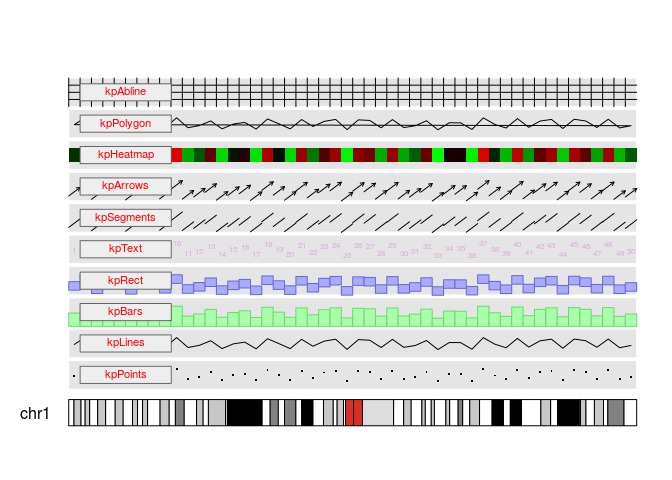## 4.3 Higher Level Plotting Functions

Higher level functions are designed to plot specific data types and objects and they expect data to be given using a specific object.

These are the currently available high-level plotting functions:

• kpPlotRegions Accepts a GRanges object and plots the regions as solid rectangles. If there are overlapping regions, it automatically detect them and plot them in a stacked way.
• kpPlotCoverage Accepts a Granges object and plots the coverage level over the genome as a histogram-like bar plot.

# 5 Compatibility with magrittr

karyoploteR is compatible with magrittr and so it is also possible to use %>% pipes to chain the calls to the plotting functions. This allows somewhat simpler code.

For example this code

  kp <- plotKaryotype(genome="hg19")
kpDataBackground(kp)
kpAxis(kp)
kpPlotRegions(kp, gains, col="#FFAACC")
kpPlotRegions(kp, losses, col="#CCFFAA")

is equivalent to this one using pipes

  library(magrittr)
kp <- plotKaryotype(genome="hg19") %>% kpDataBackground() %>%  kpAxis() %>%
kpPlotRegions(gains, col="#FFAACC") %>%
kpPlotRegions(losses, col="#CCFFAA")

# 6 Session Info

sessionInfo()
## R version 3.4.2 (2017-09-28)
## Platform: x86_64-pc-linux-gnu (64-bit)
## Running under: Ubuntu 16.04.3 LTS
##
## Matrix products: default
## BLAS: /home/biocbuild/bbs-3.5-bioc/R/lib/libRblas.so
## LAPACK: /home/biocbuild/bbs-3.5-bioc/R/lib/libRlapack.so
##
## locale:
##   LC_CTYPE=en_US.UTF-8       LC_NUMERIC=C
##   LC_TIME=en_US.UTF-8        LC_COLLATE=C
##   LC_MONETARY=en_US.UTF-8    LC_MESSAGES=en_US.UTF-8
##   LC_PAPER=en_US.UTF-8       LC_NAME=C
##  LC_MEASUREMENT=en_US.UTF-8 LC_IDENTIFICATION=C
##
## attached base packages:
##  parallel  stats4    stats     graphics  grDevices utils     datasets
##  methods   base
##
## other attached packages:
##   BSgenome.Hsapiens.UCSC.hg19_1.4.0
##   magrittr_1.5
##   karyoploteR_1.2.2
##   regioneR_1.8.1
##   BSgenome_1.44.2
##   rtracklayer_1.36.5
##   Biostrings_2.44.2
##   XVector_0.16.0
##  GenomicRanges_1.28.6
##  GenomeInfoDb_1.12.3
##  IRanges_2.10.4
##  S4Vectors_0.14.6
##  BiocGenerics_0.22.1
##  memoise_1.1.0
##  knitr_1.17
##  BiocStyle_2.4.1
##
## loaded via a namespace (and not attached):
##   Biobase_2.36.2                httr_1.3.1
##   bit64_0.9-7                   AnnotationHub_2.8.2
##   splines_3.4.2                 shiny_1.0.5
##   Formula_1.2-2                 interactiveDisplayBase_1.14.0
##   latticeExtra_0.6-28           blob_1.1.0
##  GenomeInfoDbData_0.99.0       Rsamtools_1.28.0
##  yaml_2.1.14                   RSQLite_2.0
##  backports_1.1.1               lattice_0.20-35
##  biovizBase_1.24.0             digest_0.6.12
##  RColorBrewer_1.1-2            checkmate_1.8.4
##  colorspace_1.3-2              httpuv_1.3.5
##  htmltools_0.3.6               Matrix_1.2-11
##  plyr_1.8.4                    XML_3.98-1.9
##  biomaRt_2.32.1                zlibbioc_1.22.0
##  xtable_1.8-2                  scales_0.5.0
##  BiocParallel_1.10.1           htmlTable_1.9
##  tibble_1.3.4                  AnnotationFilter_1.0.0
##  ggplot2_2.2.1                 SummarizedExperiment_1.6.5
##  GenomicFeatures_1.28.5        nnet_7.3-12
##  lazyeval_0.2.0                mime_0.5
##  survival_2.41-3               evaluate_0.10.1
##  foreign_0.8-69                BiocInstaller_1.26.1
##  tools_3.4.2                   data.table_1.10.4
##  matrixStats_0.52.2            stringr_1.2.0
##  munsell_0.4.3                 cluster_2.0.6
##  DelayedArray_0.2.7            AnnotationDbi_1.38.2
##  ensembldb_2.0.4               compiler_3.4.2
##  rlang_0.1.2                   grid_3.4.2
##  RCurl_1.95-4.8                dichromat_2.0-0
##  VariantAnnotation_1.22.3      htmlwidgets_0.9
##  bitops_1.0-6                  base64enc_0.1-3
##  rmarkdown_1.6                 gtable_0.2.0
##  curl_2.8.1                    DBI_0.7
##  R6_2.2.2                      GenomicAlignments_1.12.2
##  gridExtra_2.3                 bit_1.1-12
##  Hmisc_4.0-3                   rprojroot_1.2
##  ProtGenerics_1.8.0            stringi_1.1.5
##  Rcpp_0.12.13                  rpart_4.1-11
##  acepack_1.4.1